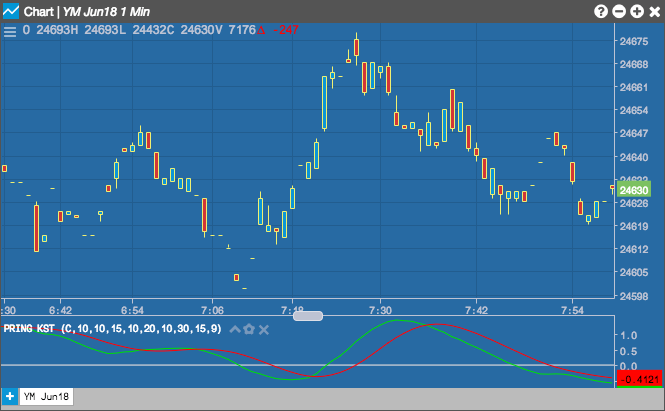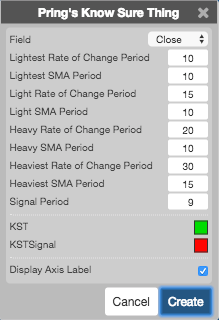Charts

# Pring's Know Sure Thing (KST)

The Pring's Know Sure Thing (KST) indicator is a momentum oscillator based on the smoothed rate-of-change for four different timeframes. Pring frequently applied trend lines to KST. Although trend line signals do not occur often, Pring notes that such breaks reinforce signal line crossovers.## Configuration Options• Field: Price or combination of prices to use as the base for average calculations. Possible values include:
• Open
• High
• Low
• Close
• HL/2 $$\left ( \frac{High + Low}{2} \right )$$
• HLC/3 $$\left ( \frac{High + Low + Close}{3} \right )$$
• HLCC/4 $$\left ( \frac{High + Low + Close + Close}{4} \right )$$
• OHLC/4 $$\left ( \frac{Open + High + Low + Close}{4} \right )$$
• Lightest Rate of Change Period: TBD
• Lightest SMA Period: TBD
• Light Rate of Change Period: TBD
• Light SMA Period: TBD
• Heavy Rate of Change Period: TBD
• Heavy SMA Period: TBD
• Heaviest Rate of Change Period: TBD
• Heaviest SMA Period: TBD
• Signal Period: TBD
• Color Selectors: Colors to use for graph elements.
• Display Axis Label: Whether to display the most recent value on the Y axis.

## Formula

The indicator formula utilizes four different time frames to show overall momentum, and not just momentum over one specific timeframe:

• ROCMA1 = 10-Period SMA of 10-Period Rate-of-Change
• ROCMA2 = 10-Period SMA of 15-Period Rate-of-Change
• ROCMA3 = 10-Period SMA of 20-Period Rate-of-Change
• ROCMA4 = 15-Period SMA of 30-Period Rate-of-Change

$KST Line = (RoCMA1 \times 1) + (RoCMA2 \times 2) + (RoCMA3 \times 3) + (RoCMA4 \times 4)$

Signal Line = 9-period SMA of KST

where, SMA stands for Simple Moving Average and ROC stands for Rate-of-Change.